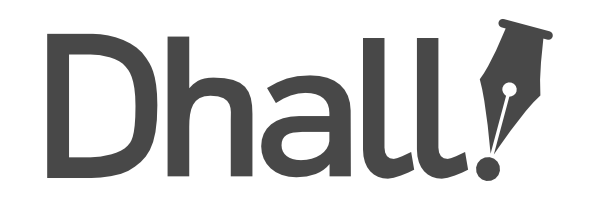# Dhall as an alternative to OpenAPI/GraphQL

#1

I’ve seen this issue come up several times in the past, but I had time this weekend to play around with the idea and came up with something. I made a gist and wrote this short article about it: https://dev.to/meeshkan/reviving-the-dhall-api-discussion-10k0. Curious to hear your thoughts - please post them either in the article or on this thread!

#2

I am not sure I understood correctly, is the client workflow supposed to first send a token and get a json schema in return, and then send a Projection Type and ProjectionF function to get the Projection back?

It’s a bit confusing to see a json schema in the middle, shouldn’t that be a Dhall schema instead?

#3

That’s the idea.

The reason it is JSON schema (https://json-schema.org/) is because dhall cannot encode recursive relationships between objects with the same ease as JSON schema. In the example I gave, owners have pets and every pet has an owner.

The JSON schema only needs to be fetched once (like a graphql schema), and the nonce is a way for the server to verify that the projection function is up to date.

#4

Yeah, the lack of language support for recursive types is going to be a major issue.

Another issue I can foresee is the inability to specify things like minimum/maximum bounds or regular expression patterns.

One other thing to note is that `Reader` is isomorphic to `Db`, so you can simplify the code by replacing all occurrences of `Reader` with `Db`, like this:

``````let fold = https://prelude.dhall-lang.org/List/fold

let filter = https://prelude.dhall-lang.org/List/filter

let equal = https://prelude.dhall-lang.org/Natural/equal

let Obj = < User | Pet | List >

let Key = < Id | Type | Name | Pets | Head | Tail >

let Entry =
< Int_ : Integer
| Bool_ : Bool
| Double_ : Double
| Text_ : Text
| Id_ : Natural
| Type_ : Obj
| Nil
>

let Row = { ptr : Natural, key : Key, val : Entry }

let Db = List Row

= [ { ptr = 0, key = Key.Id, val = Entry.Id_ 0 }
, { ptr = 0, key = Key.Type, val = Entry.Type_ Obj.User }
, { ptr = 0, key = Key.Name, val = Entry.Text_ "Mike" }
, { ptr = 0, key = Key.Pets, val = Entry.Id_ 1 }
, { ptr = 1, key = Key.Type, val = Entry.Type_ Obj.List }
, { ptr = 1, key = Key.Head, val = Entry.Id_ 10 }
, { ptr = 1, key = Key.Tail, val = Entry.Nil }
, { ptr = 10, key = Key.Id, val = Entry.Id_ 10 }
, { ptr = 10, key = Key.Type, val = Entry.Type_ Obj.Pet }
, { ptr = 10, key = Key.Name, val = Entry.Text_ "Fluffy" }
]

let getName
: Db → Natural → Text
= λ(db : Db) →
λ(ptr : Natural) →
fold
Row
(filter Row (λ(a : Row) → equal a.ptr ptr) db)
Text
( λ(a : Row) →
λ(t : Text) →
merge
{ Id = t
, Type = t
, Name =
merge
{ Int_ = λ(v : Integer) → t
, Bool_ = λ(v : Bool) → t
, Double_ = λ(v : Double) → t
, Text_ = λ(v : Text) → v
, Id_ = λ(v : Natural) → t
, Type_ = λ(v : Obj) → t
, Nil = t
}
a.val
, Pets = t
, Tail = t
}
a.key
)
""

let View = { name : Text }

in  { name = getName ReaderImpl 0 }
``````

#5

Thanks for the code simplifications! The reason I did it that way was to create a model where mutations and queries could be chained together. So the writer would be:

``````let Writer: Type = forall (Projection: Type)
-> forall (TransformF: Db -> Db)
-> forall (ProjectionF: Db -> Projection)
-> Projection
``````

The goal of that is to be able to extract info that someone is reading/writing from the haskell syntax tree (see below).

I find the lack of recursive types really compelling - recursive types are useful in a spec, but no request to a server should result in a recursive loop, and using dhall-lang to construct an executor guarantees that.

Re string matching and bounds, it falls into the general problem of comonadic patterns where you are given the world and have to extract a value. I’m thinking of Facebook’s search bar, for example - it’s not a practical strategy for `ReaderImpl` to contain all Facebook users so that a person can filter by name and age and return 10 of them.

My (still half baked) idea is to use `dhall-haskell-syntax-tree` to fish out the query, parse that, and feed it to an interpreter that does the actual data fetching. This is why I found stuff like `ReaderImpl` and `WriterImpl` compelling, although there are probably shortcomings with that approach that I’m not seeing. Ie the output for `ReaderImpl` in my example is:

``````  ( App
( Var ( V "ReaderImpl" 0 ) )
( Var ( V "View" 0 ) )
)
( Lam "db"
( Var ( V "Db" 0 ) )
( RecordLit
( fromList
[
( "name"
, App
( App
( Var ( V "getName" 0 ) )
( Var ( V "db" 0 ) )
) ( NaturalLit 0 )
)
]
)
)
)
``````

Also, for string matching, I saw a great talk by Liran Tal at Snyk about the downsides of using regexes and how they open up several vectors of attack. Currently, Dhall folds over lists containing decimal representations of unicode characters can everything that `LIKE` in SQL can do.

Thanks again for your ideas, if/when I have time I’ll try to hack at this more, and if you have more ideas please let me know!